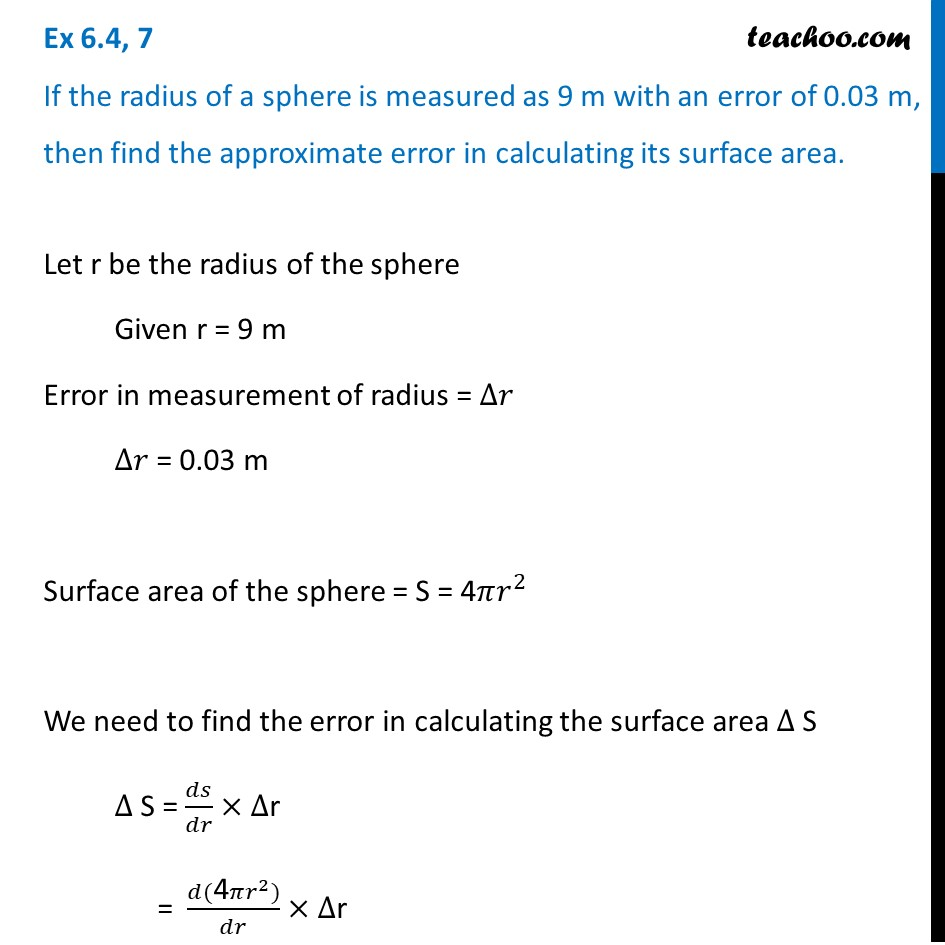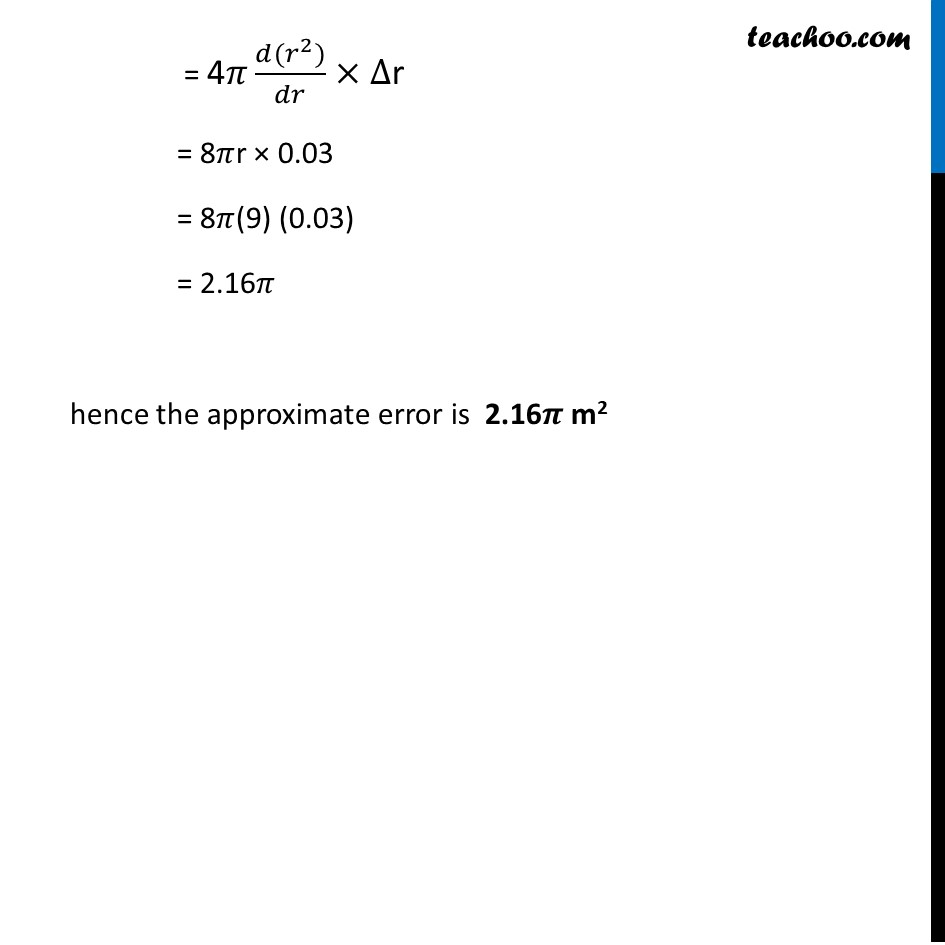Approximations (using Differentiation)

Chapter 6 Class 12 Application of Derivatives
Serial order wiseLearn in your speed, with individual attention - Teachoo Maths 1-on-1 Class

### Transcript

Question 7 If the radius of a sphere is measured as 9 m with an error of 0.03 m, then find the approximate error in calculating its surface area.Let r be the radius of the sphere Given r = 9 m Error in measurement of radius = ∆𝑟 ∆𝑟 = 0.03 m Surface area of the sphere = S = 4𝜋𝑟^2 We need to find the error in calculating the surface area ∆ S ∆ S = 𝑑𝑠/𝑑𝑟×∆"r" = (𝑑("4" 𝜋𝑟^2))/𝑑𝑟×∆"r" = "4" 𝜋 (𝑑(𝑟^2))/𝑑𝑟×∆"r" = 8𝜋r × 0.03 = 8𝜋(9) (0.03) = 2.16𝜋 hence the approximate error is 2.16𝝅 m2